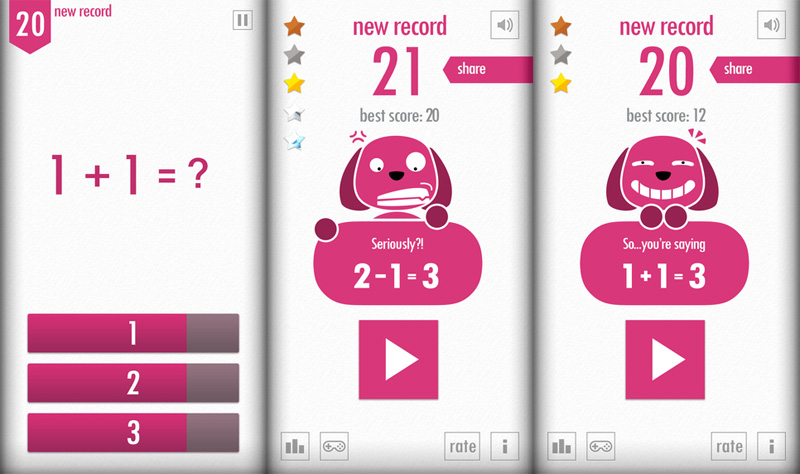# 1+2=3 HTML5 game made in 100 lines of code – brackets included – using Phaser

There are fun and addictive games which seem really easy to code, and in most cases they are.

This is the case of 1+2=3 game for iOS. This is a very simple math game which all the questions and answers are combined by 1, 2, 3, plus and minus. You just have to tell the result of some simple sums like 1+1, 2+1, 3-2 and so on. You will always be using only 1, 2 and 3.I tried to make the game in 100 lines of code, brackets included and with a correct indentation, and that’s what I got:

```window.onload = function() {
var game = new Phaser.Game(500, 500, Phaser.CANVAS, "", {
create: onCreate
});
var sumsArray = [];
var questionText;
var randomSum;
var timeTween;
var numberTimer;
var score=0;
var scoreText;
var isGameOver = false;
var topScore;
var numbersArray = [-3,-2,-1,1,2,3];
function buildThrees(initialNummber,currentIndex,limit,currentString){
for(var i=0;i0 && sum<4 && currentIndex==limit){
sumsArray[limit][sum-1].push(outputString);
}
if(currentIndex0) {
timeTween.stop();
}
gameOver(button.frame+1);
}
}
}
function nextNumber(){
scoreText.text = "Score: "+score.toString()+"\nBest Score: "+topScore.toString();
game.tweens.removeAll();
}
if(score>0){
timeTween.to({
x: -350
}, 3000, "Linear",true);
gameOver("?");
}, this);
}
randomSum = game.rnd.between(0,2);
questionText.text = sumsArray[Math.min(Math.round((score-100)/400)+1,4)][randomSum][game.rnd.between(0,sumsArray[Math.min(Math.round((score-100)/400)+1,4)][randomSum].length-1)];
}
}```

Using Phaser was really easy to create the game, and the only difficult thing – considering the 100 lines limit – was to find an algorithm to generate the random questions. I tried in various ways and eventually ended with a dirty trick.

Here is the game:

Click the button with 1, 2 or 3 according to the expression shown. When it’s game over, reload the game. What’s your best score?

214 GAME PROTOTYPES EXPLAINED WITH SOURCE CODE
// 1+2=3
// 10000000
// 2 Cars
// 2048
// Avoider
// Ballz
// Block it
// Blockage
// Bloons
// Boids
// Bombuzal
// Breakout
// Bricks
// Columns
// CubesOut
// Dots
// DROP'd
// Dudeski
// Eskiv
// Filler
// Fling
// Globe
// HookPod
// Hundreds
// InkTd
// Iromeku
// Lumines
// Magick
// MagOrMin
// Maze
// Memdot
// Nano War
// Nodes
// o:anquan
// Ononmin
// Pacco
// Phyballs
// Platform
// Poker
// Pool
// Poux
// Pudi
// qomp
// Racing
// Renju
// SameGame
// Security
// Sling
// Slingy
// Sokoban
// Splitter
// Sproing
// Stack
// Stringy
// Sudoku
// Tetris
// Threes
// Toony
// Turn
// TwinSpin
// vvvvvv
// Wordle
// Worms
// Yanga
// Zhed
// zNumbers